# Financial Functions

Financial functions are used for various financial calculations involving interest rates, loan terms, present values, and future values. Financial functions are essential for performing in-depth financial analysis of purchases, investments, and cash flow.

#### DDB

The DDB function calculates depreciation using the double declining balance method, which accelerates the rate of depreciation early in the life of an asset. When the asset's book value depreciates to the salvage value, depreciation stops.

The basic format for the DDB function looks like this:

` DDB(cost,salvage,life,period,factor) `

Enter values ( cell references or constant values) for the initial cost , the salvage value, the life of the asset, and the period for which depreciation is being calculated. The life and period should both be in the same time measurement, such as years , months, or days. Optionally, you can enter a factor for the rate of depreciation. If you do not enter a factor , the DDB function assumes a factor of 2 (that is, double declining).

For example, suppose you are depreciating an asset over a 10-year life and want to calculate the depreciation in year 10. The cost ( 10,000 ) is in cell A5, and the salvage value ( 500 ) is in cell A6. The formula with the DDB function and the result of the calculation is

` =DDB(A5,A6,10,10)    268.44 `

#### FV

FV is the future value. The syntax for the FV function looks like this:

` =FV(interest rate,periods,payment amount,present value,type) `

FV calculates the future value of an investment after payments have been made at a particular rate over a particular amount of time. The function finds the total dollar value of an investment after the investment has matured. Enter the interest rate as the periodic rate (usually a monthly or yearly rate). The periods argument contains the number of periods that the investment is active. You should use the same type of period for this argument and the interest rate (in months, years, and so on). The payment amount is the amount of each payment to the investment and represents a regular amount. The present value is the starting value of the investment. The type indicates whether payments are made at the beginning or end of the period. Entering 1 indicates the beginning and indicates the end. Of course, any of these arguments can be cell references.

Remember that cash paid out must be shown as a negative number, and interest or cash received is a positive number. Therefore, if you are calculating the future value of a savings account, the present value (amount deposited) and the payment amount (monthly deposits) are negative numbers because they are "paid out" to the investment.

Consider the following example of a formula with the FV function and its result:

` =FV(12%/12,120,-125,0, 1)    29042.38 `

Notice that the annual interest rate is converted to a monthly (periodic) rate using the formula 12%/12 and that the term and payment values reflect the same type of period (months). The result shows that you will have \$29,042.38 after 10 years (120 months) of depositing \$125 monthly at a 12% annual interest rate.

#### IPMT

This financial function calculates the interest paid for a particular payment given the interest rate , the number of periods in the term, and the present value . Specify the period for which you want to determine the interest being paid. The type determines whether payments are made at the beginning ( 1 ) of the period or at the end ( ). The syntax for the IPMT function is

` =IPMT(rate,period,periods,present value,future value,type) `

rate is the interest rate per period. period is the period for which you want to find the interest, and must be in the range 1 to nper . periods is the total number of payment periods in an annuity. present value is the lump-sum amount that a series of future payments is worth right now. The future value is a cash balance you want to attain after the last payment is made. If you omit the future value, Excel assumes the future value is . The future value of a loan, for example, is . type is the number 0 or 1 and indicates when payments are due. If you omit the type, Excel assumes the type is . If payments are due at the end of the period, set the type to . If the payments are due at the beginning of the period, set the type to 1 .

Make sure that you are consistent about the units you use for specifying rate and periods. For instance, if you make monthly payments on a three-year loan at 10% annual interest, use 10%/12 for rate and 3*12 for nper . If you make annual payments on the same loan, use 10% for rate and 3 for nper . For all the arguments, cash you pay out, such as deposits to savings, is represented by negative numbers. Cash you receive, such as dividend checks, is represented by positive numbers.

For example, the following formula calculates the interest due in the first month of a three-year, \$10,000 loan at 10% annual interest:

` =IPMT(0.1/12,1,36,10000) `

The interest due in the first month of the loan is \$83.33.

#### NPER

NPER calculates the number of required pay periods for an investment based on periodic, constant payments, and a constant interest rate. The syntax for the NPER financial function is

` =NPER(rate,payment,present value,future value,type) `

rate is the interest rate per period. payment is the amount of each payment to the investment and represents a regular amount. present value is the lump-sum amount that a series of future payments is worth right now. The future value is a cash balance you want to attain after the last payment is made. If you omit the future value, Excel assumes the future value is . The future value of a loan, for example, is . type is the number or 1 and indicates when payments are due. If you omit the type, Excel assumes the type is . If payments are due at the end of the period, set the type to . If the payments are due at the beginning of the period, set the type to 1 .

For example, suppose you want to buy a boat. The cost of the boat is \$4,500, and the bank will loan you the amount. You know you can afford to pay \$125.00 at the beginning of each month if you borrow the money. How many monthly payments will you have to make to pay off a \$4,500 loan at 8% yearly interest? The following NPER function will calculate the number of monthly payments:

` =NPER(0.08/12,-125,4500,0,1) `

The number of monthly payments is 40.9, and rounded up, is 41.

#### NPV

The NPV function calculates the net present value of a series of cash-flow transactions or an investment based on a series of periodic cash flows and a discount rate. rate is the periodic interest rate of an investment of equivalent risk, and the range is the range of cells containing the cash incomes or outflows. If you are the investor or lender, remember that the loan paid out is negative and the payments in are positive. If the result is a positive number, the investment can be considered a good one. The basic format of the NPV function is

` =NPV(rate,range) `

What if you consider an investment in which you pay \$10,000 one year from today and receive an annual income of \$3,000, \$4,200, and \$6,800 in the next three years. Assuming an annual discount rate of 10%, the net present value of this investment is

` NPV(10%,-10000,3000,4200,6800) `

The net present value is \$1,188.44.

#### PMT

The PMT function calculates the periodic payment when you enter the interest rate , periods , and principal as arguments:

` =PMT(rate,periods,principal) `

The following To Do exercise shows you how to set up and use loan calculations. Excel provides a number of functions for calculating loan amounts. There are four parts of a loan: the principal amount, periodic interest rate, periodic payment, and number of payments (or term).

The purpose of the worksheet is to calculate any one of these amounts when you know the other three. For example, you can calculate the monthly payment amount when you know the principal, interest rate, and term. You'll be entering data in a blank worksheet to prepare for calculating the monthly payment.

##### To Do: Calculate the Monthly Payment
1. Enter data into a new worksheet to match the data in the worksheet shown in Figure 15.3. For the interest amount, be sure to type 0.6 and to format the number with the Percent Style tool on the Formatting toolbar.

##### Figure 15.3. The beginning of a loan-calculation worksheet.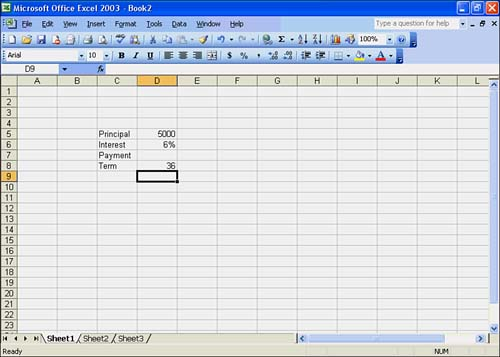2. Suppose you want to calculate the monthly payment amount using the worksheet from Figure 15.3. Simply enter the formula =PMT(D5/12,D7,D4) into cell D6 as shown in Figure 15.4. In this case, you would enter values for the interest rate, periods, and principal only.

##### Figure 15.4. Example of calculating the payment of a loan.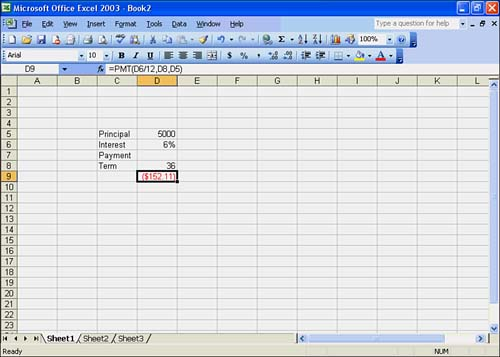Notice that the interest rate is entered as the periodic rate. This periodic rate should be for the same time periods entered in the periods argument. In the example, the term is 36 months (36 periods ), so you've entered the monthly interest rate. If you know only the annual interest rate, simply divide the annual rate by 12 to get the monthly rate. Or you can enter the formula =12%/12 to calculate the periodic rate from the annual rate.

The result in cell D6 should be (\$152.11) . The formula in the Formula bar should read =PMT(D6/12,D8,D5) . Notice that the payment amount appears as a negative because it represents cash out. When using sums you have borrowed, the principal is positive (cash in) and the payment is negative (cash out). For sums loaned, the principal is negative and the payment is positive.

#### PPMT

The PPMT function calculates the amount of principal being paid during any of the payment periods, given the periodic interest rate and number of periods. A complete example of using this function to view the principal amount of each payment appears later in this section. The PPMT function looks like this:

` =PPMT(rate,period,periods,present value,future value,type) `

Given the rate , period , and principal , PPMT calculates the principal payment for any given payment number. The format for the PPMT function is

` =PPMT(rate,payment number,periods,principal) `

Follow the steps in the To Do exercise to calculate the amount of interest and principal paid at each payment. As you might already know, you pay more interest at the beginning of a loan than at the end. The proportion of interest to principal is different for each payment. You can use the PPMT function to determine how much principal is being paid with each payment. You start by entering data in the same worksheet used in the previous exercise in preparation for using the PPMT function.

##### To Do: Calculate How Much Principal Is Paid with Each Payment
1. Enter the heading, column headings, and payment numbers from 1 to 36 (or whatever the term requires) down column C, as shown in Figure 15.5. Figure 15.5 also shows some basic formatting applied to the worksheet, including the border around the table of values and some number formats.

##### Figure 15.5. Enter the payment numbers on the worksheet.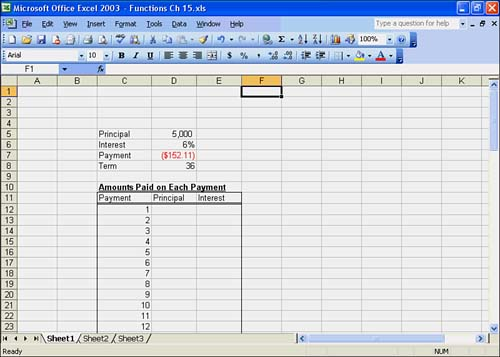2. The first formula calculates the interest paid at the first payment. In cell D12, enter the formula =PPMT(\$D\$6,C12,\$D\$8,\$D\$5) .

The result should be (\$41.97) . Notice that all the references are absolute except the payment number, which is copied down column D to produce each payment.

3. Enter the formula =\$D\$7-D12 for the interest amount in cell E12.

This formula simply takes the payment amount and subtracts the PPMT amount for each payment number. You can see that the first payment consists of \$41.97 of principal and \$110.14 of interest.

4. To view the remaining payments, select D12:E12 and use the fill handle to fill the range D13:E47. The result should look something like Figure 15.6.

##### Figure 15.6. Payment formulas.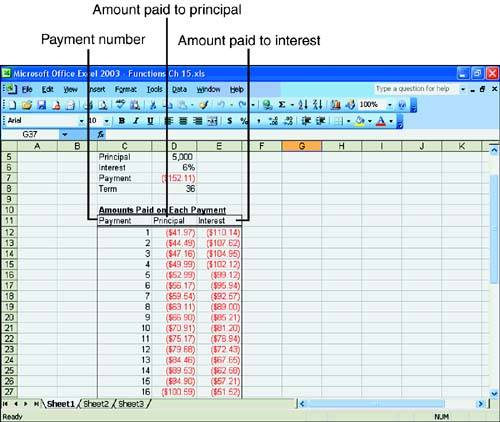5. Use the SUM function to total each column at the bottom.

6. Add the two totals for the entire amount of the loan, including principal and interest. The totals are shown in Figure 15.7.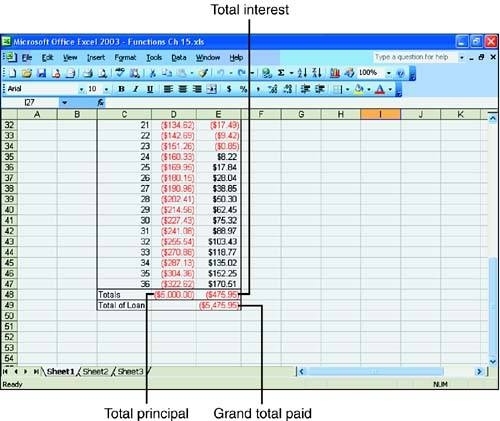#### RATE

The RATE function calculates the interest rate or discount rate for a loan or investment when you enter the term , payment , and principal as arguments:

` =RATE(term,payment,principal) `

An example of using the RATE financial function is to calculate the rate of a four-year \$8,000 loan with monthly payments of \$200. Here's the formula:

` =RATE(48, -200, 8000) `

The result is a rate of 0.77%. This is the monthly rate, because the period is monthly. The annual rate is 0.77%*12, which equals 9.24%.

#### SLN

The SLN function returns the straight-line depreciation of an asset for one period. This method accelerates depreciation at a constant rate for the entire life of the asset. Enter the initial cost , the salvage value, and the life of the asset (in years). cost is the initial cost of the asset. salvage is the value of the asset at the end of the depreciation. Life is the number of periods over which the asset is being depreciated. The syntax of an SLN function looks like the following:

` =SLN(cost,salvage,life) `

An example of the SLN function is calculating the straight-line depreciation for a truck that costs \$30,000, which has a useful life of 10 years and a salvage value of \$7,500. The SLN formula would look like this:

` =SLN(30000,7500,10) `

The result is a straight-line depreciation of \$2,250.

#### SYD

The SYD function is a third depreciation method called the sum of the years' digits method. Enter the initial cost , the salvage value, the life of the asset, and the period for which depreciation is calculated. The SYD function format has this syntax:

` =SYD(cost,salvage,life,period) `

You can use the SYD function to calculate depreciation in year 10 when the asset has a 10-year life, cell A5 contains 10,000 , and cell A6 contains 500 . The formula and its result are as follows :

` =SYD(A5,A6,10,10)    172.73 `Sams Teach Yourself Microsoft Office Excel 2003 in 24 Hours (Sams Teach Yourself in 24 Hours)
ISBN: 1435276337
EAN: 2147483647
Year: 2003
Pages: 279
Authors: Trudi Reisner